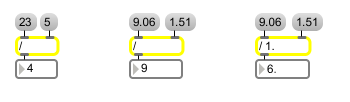# /

Divide two numbers, output the result

## Description

/ takes any two given numbers, divides them (according to the specified divisor assignment), and then outputs the result.

## Arguments

Name Type Opt Description
initial-divisor int or float opt Sets an initial value for the divisor. If there is no argument, the divisor is set to 1 initially. A float argument causes the numbers to be divided as floats. (Division by 0 is not allowed. Int division by 0 will have the same result as dividing by 1. Float division by 0 will always cause an output of -2 31 .)

## Messages

 bang In left inlet: Performs the division with the numbers currently stored. int input [int] In left inlet: The number is divided by the number in the right inlet, and the result is sent out the outlet. (inlet1) divisor [int] In right inlet: The number is stored as the divisor (the number to be divided into the number in the left inlet). float input [float] Converted to int, unless / has a float argument. set input [int] Sets the number to be divided without causing output (bang will output it). list input and divisor [list] In left inlet: The first number is divided by the second number, and the result is sent out the outlet.

## Output

float: Only if there is an argument with a decimal point.
int: The two numbers in the inlets are divided, and the result is sent out the outlet.

## ExamplesRemainder is discarded.......... Floats are truncated before division........... ......unless there is a float argument